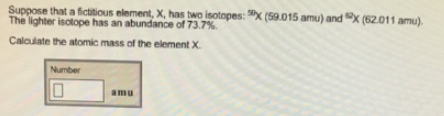Chemistry Isotopes Solution: Suppose that a fictitious element, X, has two isot...

# Solution: Suppose that a fictitious element, X, has two isotopes:  50X (59.015 amu) and 82X (62.011 amu). The lighter isotope has an abundance of 73.7%. Calculate the atomic mass of the element X.

Problem

Suppose that a fictitious element, X, has two isotopes:  50X (59.015 amu) and 82X (62.011 amu). The lighter isotope has an abundance of 73.7%.

Calculate the atomic mass of the element X.View Complete Written Solution